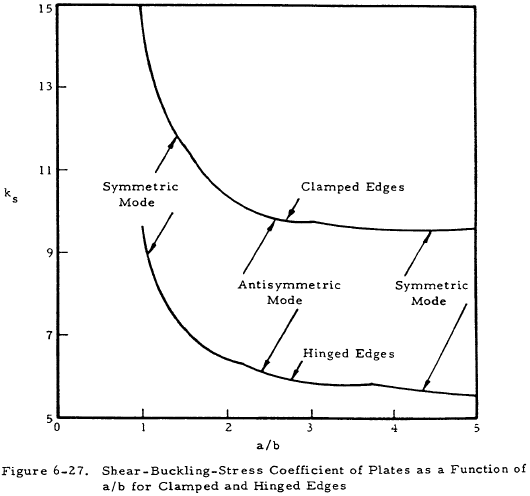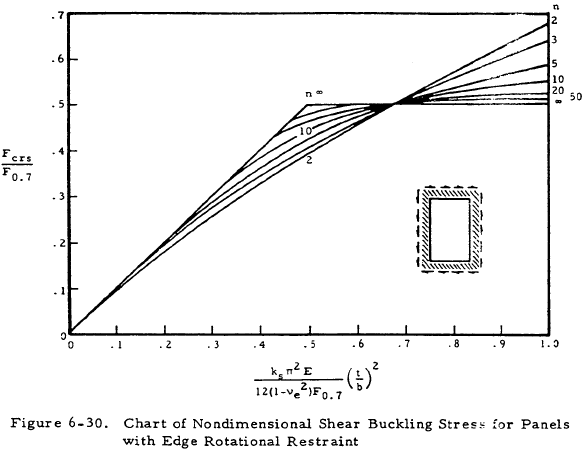﻿ Shear Buckling of Flat Plates | Engineering Library

# Shear Buckling of Flat Plates

This page provides the chapters on shear buckling of flat plates from the "Stress Analysis Manual," Air Force Flight Dynamics Laboratory, October 1986.

Other related chapters from the Air Force "Stress Analysis Manual" can be seen to the right.

Analysis of Plates

## Nomenclature

 a = plate length b = plate width E = modulus of elasticity Es = secant modulus Et = tangent modulus f = ratio of cladding thickness to total plate thickness F0.7, F0.85 = secant yield stress at 0.7E and 0.85E Fcrs = critical shear stress Fpl = stress at proportional limit ks = shear buckling coefficient
 n = shape parameter, number of half waves in buckled plate ss = simply supported t = thickness W = total load, potential energy β = ratio of cladding yield stress to core stress ϵ = ratio of rotational rigidity of plate edge stiffeners η = plasticity reduction factor η = cladding reduction factor λ = buckle half wavelength ν = inelastic Poisson's ratio νe = elastic Poisson's ratio

## 6.5 Shear Buckling of Flat Plates

The critical shear-buckling stress of flat plates may be found from Equation (6-27).

$$F_{crs} = \eta ~\overline{\eta} ~{ k_s ~\pi^2 E \over 12 (1 - \nu_e)^2 } \left({t \over b}\right)^2$$
(6-27)

Figure 6-27 presents the shear coefficient ks, as a function of the size ratio a/b for clamped and hinged edges. For infinitely long plates, Figure 6-28 presents ks as a function of λ/b. Figure 6-29(a) presents ks∞ for long plates as a function of edge restraint, and Figure 6-29(b) gives ks∞ as a function of b/a, thus allowing the determination of ks.

The nondimensional chart in Figure 6-30 allows the calculation of inelastic shear buckling stresses if the secant yield stress, F0.7, and n the shape parameter is known (Table 6-11).

The plasticity-reduction factor η and the clodding factor η can be obtained from Equations (6-28), (6-29), and (6-30).

$$\eta = { E_s \over E } \left({ 1 - \nu_e^2 \over 1 - \nu^2 }\right)$$
(6-28)
$$\eta = { 1 + 3 f \beta \over 1 + 3 f } ~\text{ for } F_{c1} \lt F_{crs} \lt F_{pl}$$
(6-29)
$$\eta = {1 \over 1+3f} { \left[1 + 3f \left( { \overline{E_s} \over E_s } \right) \right] + \left\{ \left[1 + 3f \left( { \overline{E_s} \over E_s } \right) \right] \left[ {1 \over 4} + {3 \over 4} \left( E_t \over E_s \right) + W \right] \right\}^{1/2} \over 1 + \left[ {1 \over 4} + {3 \over 4} \left( E_t \over E_s \right) \right]^{1/2} }$$
(6-30)

for Fcrs > FplTable 6-11: Values of Shape Parameter n for Several Engineering Materials
n Material
3 One-fourth hard to full hard 18-8 stainless steel
One-fourth hard 18-8 stainless steel, cross grain
5 One-half hard and three-fourths hard 18-8 stainless steel, cross grain
10 Full hard 18-8 stainless steel, cross grain
2024-T and 7075-T aluminum-alloy sheet and extrusion
2024R-T aluminum-alloy sheet
20 to 25 2024-T80, 2024-T81, and 2024-T86 aluminum alloy sheet
2024-T aluminum-alloy extrusion
SAE 4130 steel heat-treated up to 100,000 psi ultimate stress
2014-T aluminum-alloy extrusions
SAE 4130 steel heat-treated above 125,000 psi ultimate stress